Categories

# Word2Vec pre-training vector word

introduction

In many natural language processing tasks, many words is determined by the expression of their tf-idf score. Even if these scores tell us the relative importance of a word in a text, but they did not tell us the semantics of the word. Word2Vec is a Neural Network Model – In the case of a given no tag corpus, a corpus of word generating a vector capable of expressing semantics.

word2vec is a Google open source tools for word vector calculations, it can be a good measure of similarity between the word and the word;

word2vec modeling refers to the use CBoW Skip-gram model or a different model to calculate a vector of words (word vector)

CBoW a given context to predict the target word, Skip-gram context of a given input word prediction, but eventually will give “word vector matrix W” of the FIG.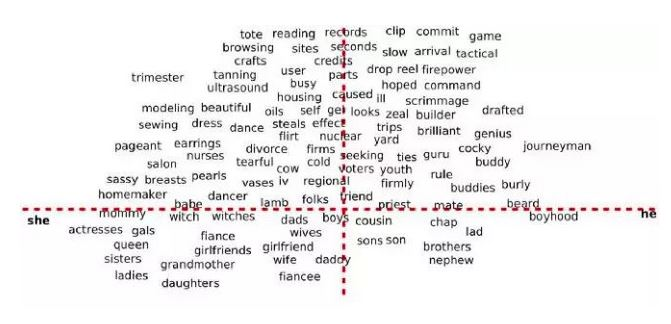Statistical Language Model (statistical language models)

Before diving word2vec, first reviewed a fundamental problem in nlp: how to calculate the probability of a text sequence appears in a certain language? (Ngram)

Statistical language model gives a basic framework to address this kind of problem. For a text sequence: its probability can be expressed as: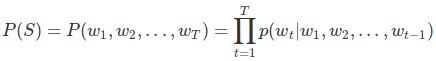Upcoming joint probability sequences into the product of a series of conditional probabilities. The question becomes how to predict the probability of a given condition under these previous words: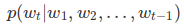Because of its huge parameter space, such an original model in practice and there is no use. We are using more simplified version –Ngram model: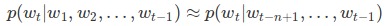The common bigram model (N = 2) and tirgram Model (N = 3). In fact, due to the complexity of the model and prediction accuracy, we rarely consider N> 3 model. We can use the maximum likelihood method to solve the parameters Ngram model – equivalent to the word frequency statistics conditions of each Ngram. To avoid the problem of probability 0 (some never appeared in the training set the probability of fragments would make the whole sequence Ngram 0) appear in the statistics, the further development of the original people of the Ngram model based on a back-off trigram model (with low-level 0 unigram and bigram probability trigram’s place) and interpolated trigram model (expressed as the conditional probability unigram, bigram, trigram three linear function).

Distributed Representation (represented semantic distribution)

However, there are still limitations Ngram model. First word sequence can not deal with N> 3 of (practical application of> 3 will be explosive growth in the parameter space, the curse of dimensionality problem), and secondly it does not take into account the intrinsic link between the word and the word (just probability of word frequency statistics) . For example, consider “the cat is walking in the bedroom” this sentence. If we see a lot in the training corpus similar to “the dog is walking in the bedroom” or “the cat is running in the bedroom” such a sentence, so even if we have not seen this sentence, also from the “cat similarity between “and” dog “(” walking “and” running “), speculated that the probability of this sentence. However, Ngram model can not.

So, people will naturally think, whether with a continuous dense vector to describe the features of a word it? So we can go not only portray the similarity between the word and the word can also create a smooth function of the probability vector model, makes similar words vector can be mapped to a similar probability space. This vector is also referred to as a dense continuous word is distributed representation (Distributed shown).

In fact, this concept in information retrieval (Information Retrieval) areas have long been widely used. Only in the IR field, this concept is known as a vector space model (Vector Space Model, hereinafter referred to as VSM).

VSM is a Statistical Semantics Hypothesis: Statistical information hidden features of the language semantics (Statistical pattern of human word usage can be used to figure out what people mean). For example, two words with a similar document distribution can be considered with a similar theme. The Hypothesis There are a lot of derivative versions. Among them, two better-known version is the Bag of Words Hypothesis and Distributional Hypothesis. The former is said that a document word frequency (instead of word order) represent the theme of the document; the latter is to say, two words similar context has similar semantics. Later we will see word2vec algorithm is based on the assumption of Distributional.

So, VSM is how sparse discrete one-hot word vector mapping is dense continuous Distributional Representation of it?

Briefly, based Bag of Words Hypothesis (assuming bag of words), we can construct a term-document (correlation matrix) A: matrix Ai ,: row corresponds to a dictionary word, columns of the matrix A:, J corresponds to a document where the training is expected; in the matrix element Ai, j represents the number of times word wi appear in the document Dj (or frequency). Then we can extract the column vectors as semantic vector word (although, in practice, we have more of a theme as a vector file with a column vector, ie a document word frequency represents the theme of the document)

term-document incidence matrix: doc 1: new home sales top forecasts doc 2: home sales rise in july doc 3: increase in home sales in july doc 4: july new home sales rise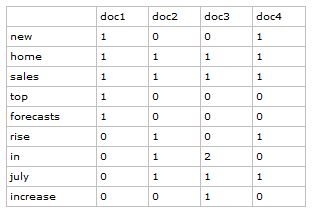Similarly, we can (expressed guess distributed feature / characteristic context shown) configured Distributional Hypothesis Based on a word-context matrix. Compared with the term-document, columns of the matrix into a context where the word, but also elements of the matrix into a number of co-occurrence of the word in a context window. word-context matrix size of a pre-specified statistical word co-occurrence frequency in the window, not only helps to bring semantic information word reflects the grammatical structure information word is still to some extent.

word-context based semantic matrix: doc1: I like deep learning doc2: I like NLP doc3: I enjoy flying window size set to 1, to obtain word-context matrix: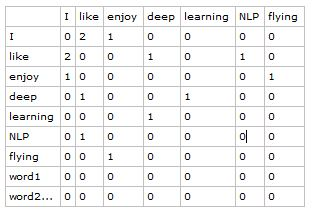Note that these two types of matrix row vectors calculated similarity with a subtle difference: term-document matrix will often given a higher similarity with a document in the two word; and word-context would matrix those two have the same context the word given a higher degree of similarity. With respect to the former which is a higher order of similarity, so it has been more widely used in conventional art information retrieval.

Neural Network Language Model (neural network model language)

Given the lack Ngram and other models, in 2003, Bengio, who published a seminal article: A neural probabilistic language model. In this article, they summed up a framework for the establishment of a statistical language model using neural networks (Neural Network Language Model, hereinafter referred to as NNLM), and the first time the word embedding the concept (though not by that name), which laid the foundation including word2vec including follow-up study of basic word representation learning.

The basic idea NNLM model can be summarized as follows:

Assuming each vocabulary word corresponds to a continuous feature vector;

Assuming a smooth continuous probability model, a period of the input sequence vector words may be output joint probability of this sequence;

At the same time the right to re-learn word vectors and probability models in the parameters.

A point worth noting is that the parameter vector word here is to learn.

In the 2003 paper, Bengio, who used a simple forward feedback neural network to fit the conditional probability of a word sequence

(Calculated Ngram probabilities). The whole network structure model shown below: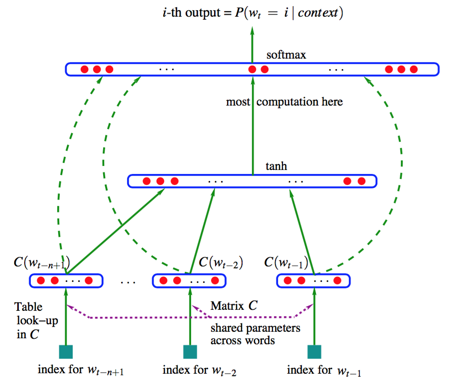We will split into two parts, the entire model to be understood:

The first is a linear Embedding layer. It will enter the N-1 word one-hot vector, shared by a matrix of D × V C, mapped to the N-1 word vector distributed (distributed vector). Wherein, V is the size of the dictionary, D is the latitude Embedding vector (a priori parameter can be adjusted). C matrix (C matrix initial value is randomly initialized) stored in the word vector to be learned.

Followed by a simple front g the feedback network. It consists of a tanh (activation function) and a hidden layer SoftMax (accounting for multiple classification output) output layer. The probability distribution vector by the N-1 output word vector mapping Embedding layer is of a length D, thereby dictionary word in the input to the conditional probability estimates in context: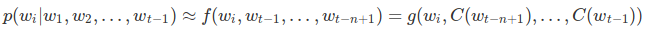We can by minimizing a cross-entropy (cross-entropy) regularization parameter θ loss function to adjust the model: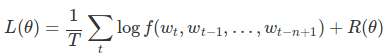Wherein the model parameter θ Embedding layer contains the elements of the matrix C, and the feedback to the neural network weight pre g in weight. This is a huge parameter space. However, in (the method of gradient descent) with SGD updating the learning parameter model, not all parameters need to be adjusted (e.g. word corresponding to the word does not appear in the context vector input). The main bottleneck in computing a normalized softmax function layer (the need for the word dictionary is calculated over all the conditional probabilities).

The following softmax function curve and calculation methods and calculation equations are given: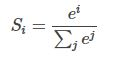∞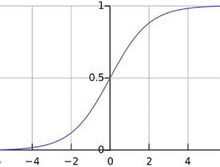∞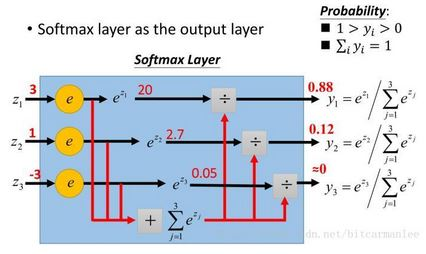(Picture from the network)

However, the abandoned complex parameter space, we must ask why such a simple model will be a huge success of it?

This model look closely you will find that it actually solve two problems at the same time: one is the calculation of the conditional probability of statistical language model in concern; one is the expression vector word vector space model in concern. The essence is not separate these two issues. We can words by introducing a continuous and smooth vector probability model space in a continuous sequence probability modeling, and thus alleviate the problem of sparsity latitude disaster data fundamentally. On the other hand, in order for the conditional probability of learning objectives to update the weight vector word heavy, with more guidance, but also coincides with the VSM in the Distributional Hypothesis.

CBoW & Skip-gram Model (word vector model algorithm)

One problem is that, like Ngram model, NNLM model can only deal with fixed-length sequence. In the 2003’s thesis, Bengio, who will model the sequence length N is capable of processing up to five, although compared bigram and trigram has been greatly improved, but still lacks flexibility. Therefore, Mikolov, who in 2010 proposed a RNNLM model, instead of the original model in the forward feedback neural network with a recurrent neural network and Embedding layer was combined with RNN in the hidden layer, thereby solving the problem of variable-length sequences .

Another problem is more serious. NNLM training too slow. Even in the order of millions of data sets, even by means of a CPU 40 for training, NNLM also need to take several weeks to give a solution to slightly fly. Obviously, for now in the hundreds of millions or even billions of real corpus, a NNLM training model is almost a impossible mission.

At this time, or that Mikolov stand out. He noted that the original NNLM training model actually can be split into two steps:

A simple model of a continuous training vector word;

Based word expression vector (vector will not train at the same time the word), a continuous training of the neural network model Ngram.

The calculation model of the main bottlenecks NNLM in the second step.

If we just want to get a continuous feature vectors word, is not it possible to simplify the second step in the neural network model it?

Mikolov think so, did the same. His breath launched two paper in 2013, and an open source tool to calculate word vector – So far, word2vec turned out, the protagonist debut.

With the front of the base, understanding word2vec becomes very simple.

First, we make the following transformation of the original NNLM model:

Before removing the non-linear feedback to the neural network hidden layer, directly softmax layer Embedding layer and output layer is connected to the intermediate layer;

Ignore the context of sequence information: All input word summary of the volume to the same Embedding layer;

The Future words into the context

The first model is a model called CBOW model (Continuous Bag-of-Words Model) is obtained, the algorithm is word2vec

You can not see the top: P

CBoW model

###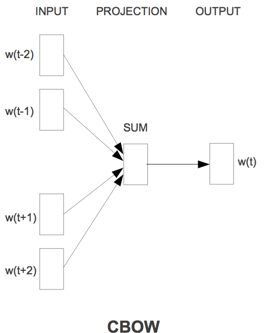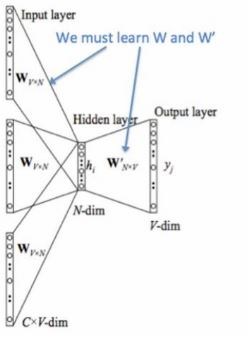Meaning two brief diagram CBOW parameters:

Input layer (Input layer): onehot context word. (Assuming word space vector of dim V, V is the number of all words in the dictionary, the word is the number of context C)

All onehot shared input respectively by weight matrix W (V * N matrix, N the number set for himself, any weight matrix W Initial value is a random initialization)

N-dim vectors is all hidden layers of the C onehot multiplied with W, and then averaging the addition, size of 1 * N

Output multiplied by a weight matrix W ‘(matrix size is N * V)

To obtain a vector (1 * V), activation function (SoftMax) treatment to give V-dim probability distribution (PS: because onehot, a dimension of each word represents the a), the maximum probability index word represented as predicted intermediate term (target word)

And the true label onehot (true label is on the left in w (t)) by comparing the error as small as possible

It is necessary to define the loss function (generally as a cross entropy cost function), gradient descent algorithm updates W and W ‘. After the training is completed, each word in the input layer and the matrix W obtained by multiplying the vector is what we want the word vector (word embedding) the W matrix (all words word embedding), also known as look up table.

Detailed example, see here

Skip-gram model

CBoW prediction model is still learning from the context of the target word to word in the expression vector. In turn, we can predict the context of the target word to word vector in learning it? The answer is yes: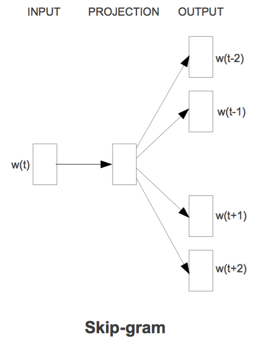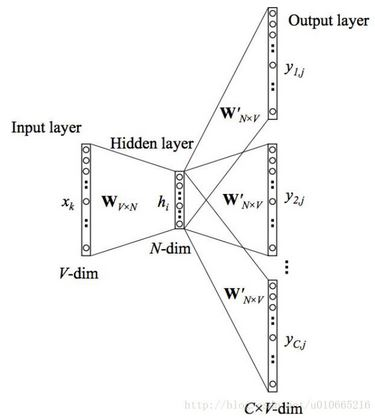This model is called Skip-gram model (derived from the name of the model will be sampled in the context of the word during training).

If the former Skip-gram model written in mathematical form (just a softmax) to the calculation, we get: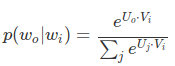Wherein, Vi Embedding layer is a column vector in the matrix, also referred to wi input vector. Uj softmax layer is the row vector in the matrix, also referred to wi output vector.

Thus, the nature of Skip-gram model is calculating the cosine similarity between the input word and the target word of input vector output vector, and softmax normalization. We should learn from the model parameters is precisely these two types of term vectors.

However, directly on the V-word dictionary and calculate the similarity normalization, and obviously a time-consuming impossible mission. To this end, Mikolov introduces two optimization algorithms: hierarchical Softmax (Hierarchical Softmax) and negative samples (Negative Sampling).

Hierarchical Softmax (level Softmax)

Softmax level approach was first introduced in 2005 by the Bengio into language model. The basic idea is complex normalized probability into a series of product in the form of conditional probabilities: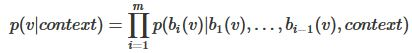Wherein each layer corresponding to the conditional probability of a binary classification problem, by a simple logic to fit the regression function. We probabilities V-word normalization issues, transformed into a probability logV words fit the problem. We can intuitively understand this process by constructing a binary tree classification. First, we have the original dictionary D is divided into two subsets D1, D2, and assuming a given context, target word probability of belonging to the subset D1 obedience logistical function of the form: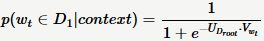Where the parameters are UDroot and Vwt model.

Next, we can subsets D1 and D2 are further divided. Repeat this process until the collection only one word. In this way, we will be the original size of the dictionary D V converted into a binary tree of depth logV. Leaf nodes of the tree with the original dictionary of word eleven correspondence; the non-leaf nodes correspond to the collection of a certain type of word. Obviously, starting from a root node to any leaf node is only one unique path – this path also encode the leaf node belongs to this category.

At the same time, starting from the root node to the leaf node process is a random walk. Therefore, we can calculate the probability of the likelihood of a binary tree leaf nodes appear Fengyun-based. For example, in the training sample for a target word wt, assuming that the corresponding coding is {1,0,1, …, 1}, we construct the likelihood function is: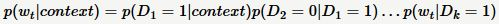We are each a function of a product of the logistic regression.

We can solve the parameters of the binary tree by maximizing the likelihood function – vector on non-leaf node, used to calculate the probability of a walk to the child node.

Softmax level is a very clever model. It will target computational complexity reduces the probability of the order logV by constructing a binary tree from the initial V to. But artificially enhanced coupling between the word and the word when the price paid. For example, changes in the conditional probability of occurrence of a word, it will affect the probability of all non-leaf nodes on the path of change, the indirect impact of the varying degrees of conditional probability other word appears. Therefore, a meaningful binary tree structure is very important. Practice has proved that, in practical applications, based on a binary tree Huffman coding to meet the needs of most application scenarios.

Negative Sampling (negative sample)

The idea originally came from a negative sample called Noise-Contrastive Estimation algorithm, originally when those parameters in order to solve the probability model can not be normalized estimate problem. And the level of the output probability transformation algorithm Softmax different model, the likelihood function when the NCE model transformation algorithm.

Skip-gram to model, for example, its original likelihood function corresponds to an Multinomial distribution. In solving this likelihood function using the maximum likelihood method, we get a cross-entropy loss function of: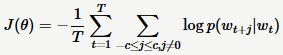Formula p (wt + j | wt) is on the whole a dictionary normalized probability.

Drive for a set of training samples , we want to know, target appeared word is from the context of driving, or a presupposed background noise: In the NCE algorithm, we construct such a problem ? Obviously, we can use a logic function to answer the question: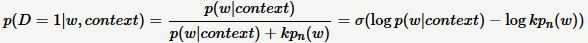This equation gives a probability of a target word w driven from the context. Wherein, k is the a priori parameter indicating the sampling frequency of the noise. p (w | context) is a non-normalized probability distribution used here molecular moiety softmax normalization function. pn (w) is the background noise word distribution. Commonly used word of unigram distribution.

By k sampling noise distribution, we get a new set of data: . Which, label marking the source (or background noise distribution of real data distribution?) Data. In this new dataset, we can come to solve the parameters of the model by maximizing the likelihood function equation logistic regression.

The negative sampling algorithm Mikolov in 2013 the thesis put forward, is a simplified version of the NCE. In this algorithm, where, Mikolov abandoned NCE likelihood function dependent on the noise-like, the direct use of the original molecule softmax function is defined in the logistic regression function further simplifies the calculations: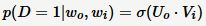In this case, the model corresponding objective function becomes: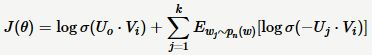In addition to optimizing algorithm level Softmax and negative samples presented here, Mikolov in 13-year paper was also introduced another trick: downsampling (subsample). The basic idea is dependent probability discard those high frequency words in training: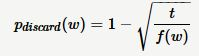Where, t is a priori parameters, and generally 10-5. F (w) w is the frequency of occurrence in the corpus. Experiments show that this down-sampling technique can significantly improve the accuracy of the word vector of the low-frequency words.

references:

.. Mikolov T, Chen K, Corrado G, et al Efficient Estimation of Word Representations in Vector Space [J] Computer Science, 2013. (article on about two models: CBOW and Skip-gram)

Mikolov T, Sutskever I, Chen K, et al Distributed Representations of Words and Phrases and their Compositionality [J] 2013, 26:… 3111-3119 (article high computational complexity for the Skip-gram model problems presented Some improvements)

Presentation on Word2Vec (NIPS 2013workshop which is on the PPT report Mikolov)

Detailed https://www.cnblogs.com/iloveai/p/word2vec.html word2vec

https://blog.csdn.net/bitcarmanlee/article/details/82320853 softmax function

https://www.jianshu.com/p/d2f0759d053c CBoW model

https://blog.csdn.net/u010665216/article/details/78721354 skip-gram function

S=w1,w2,...